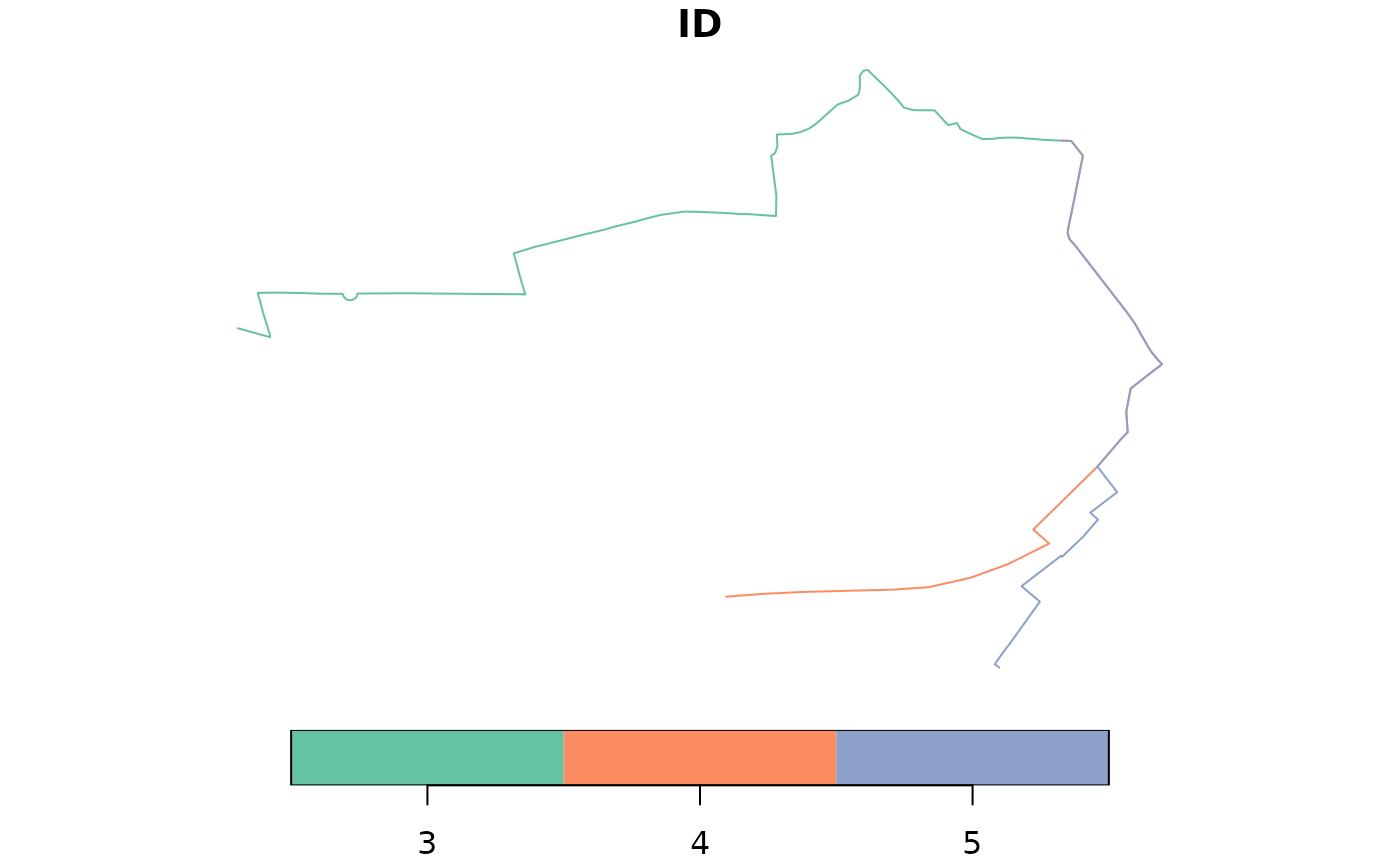Divide sf LINESTRING objects into regular segments

## Usage

``line_segment(l, n_segments, segment_length = NA)``

## Arguments

l

A spatial lines object

n_segments

The number of segments to divide the line into

segment_length

The approximate length of segments in the output (overides n_segments if set)

Other lines: `angle_diff()`, `geo_toptail()`, `is_linepoint()`, `line2df()`, `line2points()`, `line_bearing()`, `line_breakup()`, `line_midpoint()`, `line_via()`, `mats2line()`, `n_vertices()`, `onewaygeo()`, `points2line()`, `toptail_buff()`

## Examples

``````l <- routes_fast_sf[2, ]
l_seg2 <- line_segment(l = l, n_segments = 2)
#> Warning: st_linesubstring does not follow a geodesic; you may want to use st_geod_segmentize first
#> Warning: st_linesubstring does not follow a geodesic; you may want to use st_geod_segmentize first
#> Warning: st_linesubstring does not follow a geodesic; you may want to use st_geod_segmentize first
#> Warning: st_linesubstring does not follow a geodesic; you may want to use st_geod_segmentize first
plot(sf::st_geometry(l_seg2), col = 1:2, lwd = 5)``````